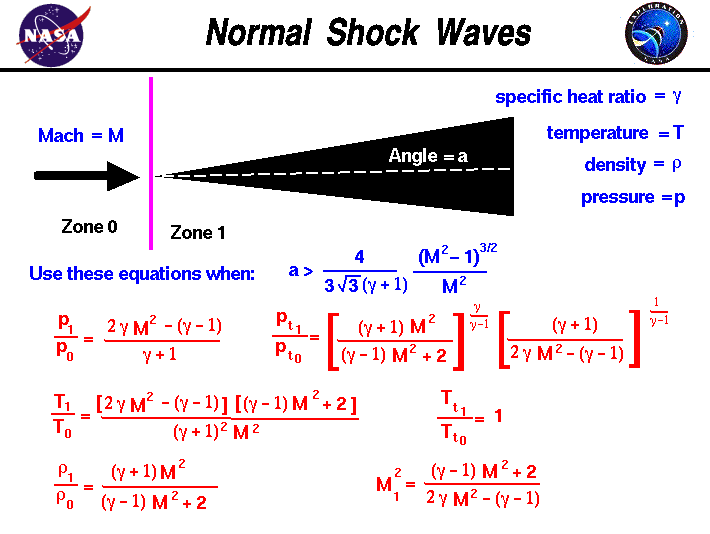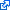+ Text Only Site
+ Non-Flash Version
+ Contact GlennAs a rocket moves through a gas, the gas molecules are deflected around the rocket. If the speed of the rocket is much less than the speed of sound of the gas, the density of the gas remains constant and the flow of gas can be described by conserving momentum and energy. As the speed of the rocket increases towards the speed of sound, we must consider compressibility effects on the gas. The density of the gas varies locally as the gas is compressed by the object. For compressible flows with little or small flow turning, the flow process is reversible and the entropy is constant. The change in flow properties are then given by the isentropic relations (isentropic means "constant entropy"). But when an object moves faster than the speed of sound, and there is an abrupt decrease in the flow area, the flow process is irreversible and the entropy increases. Shock waves are generated which are very small regions in the gas where the gas properties change by a large amount. Across a shock wave, the static pressure, temperature, and gas density increases almost instantaneously. Because a shock wave does no work, and there is no heat addition, the total enthalpy and the total temperature are constant. But because the flow is non-isentropic, the total pressure downstream of the shock is always less than the total pressure upstream of the shock; there is a loss of total pressure associated with a shock wave. The ratio of the total pressure is shown on the slide. Because total pressure changes across the shock, we can not use the usual (incompressible) form of Bernoulli's equation across the shock. The Mach number and speed of the flow also decrease across a shock wave. If the shock wave is perpendicular to the flow direction it is called a normal shock. On this slide we have listed the equations which describe the change in flow variables for flow across a normal shock. The equations presented here were derived by considering the conservation of mass, momentum, and energy. for a compressible gas while ignoring viscous effects. The equations have been further specialized for a one-dimensional flow without heat addition. The equations can be applied to the two dimensional flow past a wedge for the following combination of free stream Mach number M and wedge angle a : a > (4 / ( 3 * sqrt(3) * (gam + 1)) * {[M^2 -1]^3/2} / M^2 where gam is the ratio of specific heats. If the wedge angle is less than this detachment angle, an attached oblique shock occurs and the equations are slightly modified. Across the normal shock wave the Mach number decreases to a value specified as M1: M1^2 = [(gam - 1) * M^2 + 2] / [2 * gam * M^2 - (gam - 1)] The total temperature Tt across the shock is constant, Tt1 / Tt0 = 1 The static temperature T increases in zone 1 to become: T1 / T0 = [2 * gam * M^2 - (gam - 1)] * [(gam - 1) * M^2 + 2] / [(gam + 1)^2 * M^2] The static pressure p increases to: p1 / p0 = [2* gam * M^2 - (gam - 1)] / (gam + 1) And the density r changes by: r1 / r0 = [(gam + 1) * M^2 ] / [(gam -1 ) * M^2 + 2] The total pressure pt decreases according to: pt1 / pt0 = {[(gam + 1) * M^2 ] / [(gam - 1) *M^2 + 2]}^gam/(gam-1) * {(gam + 1) /[2 * gam * M^2 - (gam - 1)]}^1/(gam - 1) The right hand side of all these equations depend only on the free stream Mach number. So knowing the Mach number, we can determine all the conditions associated with the normal shock. The equations describing normal shocks were published in a NACA report NACA-1135 in 1951. Here's a Java program based on the normal shock equations. You can use this simulator to study the flow past a wedge. Due to IT security concerns, many users are currently experiencing problems running NASA Glenn educational applets. The applets are slowly being updated, but it is a lengthy process. If you are familiar with Java Runtime Environments (JRE), you may want to try downloading the applet and running it on an Integrated Development Environment (IDE) such as Netbeans or Eclipse. The following are tutorials for running Java applets on either IDE: NetbeansEclipseThis page shows an interactive Java applet for supersonic flow past a wedge. The applet shows the shock wave generated by the wedge and the value of the flow variables downstream of the shock. Input to the program can be made using the sliders, or input boxes at the upper right. To change the value of an input variable, simply move the slider. Or click on the input box, select and replace the old value, and hit Enter to send the new value to the program. Output from the program is displayed in output boxes at the lower right. The flow variables are presented as ratios to free stream values. The graphic at the left shows the wedge (in red) and the shock wave generated by the wedge as a line. The line is colored blue for an oblique shock and magenta when the shock is a normal shock. The black lines show the streamlines of the flow past the wedge. Notice that downstream (to the right) of the shock wave, the lines are closer together than upstream. This indicates an increase in the density of the flow. If you are an experienced user of this simulator, you can use a sleek version of the program which loads faster on your computer and does not include these instructions. You can also download your own copy of the program to run off-line by clicking on this button: Guided ToursCompressible Aerodynamics:Shock Waves:Shock Simulator:Activities: Related Sites: Rocket Index Rocket Home Beginner's Guide Home+ Inspector General Hotline + Equal Employment Opportunity Data Posted Pursuant to the No Fear Act + Budgets, Strategic Plans and Accountability Reports + Freedom of Information Act + The President's Management Agenda + NASA Privacy Statement, Disclaimer, and Accessibility CertificationEditor: Tom Benson NASA Official: Tom Benson Last Updated: May 13 2021 + Contact Glenn